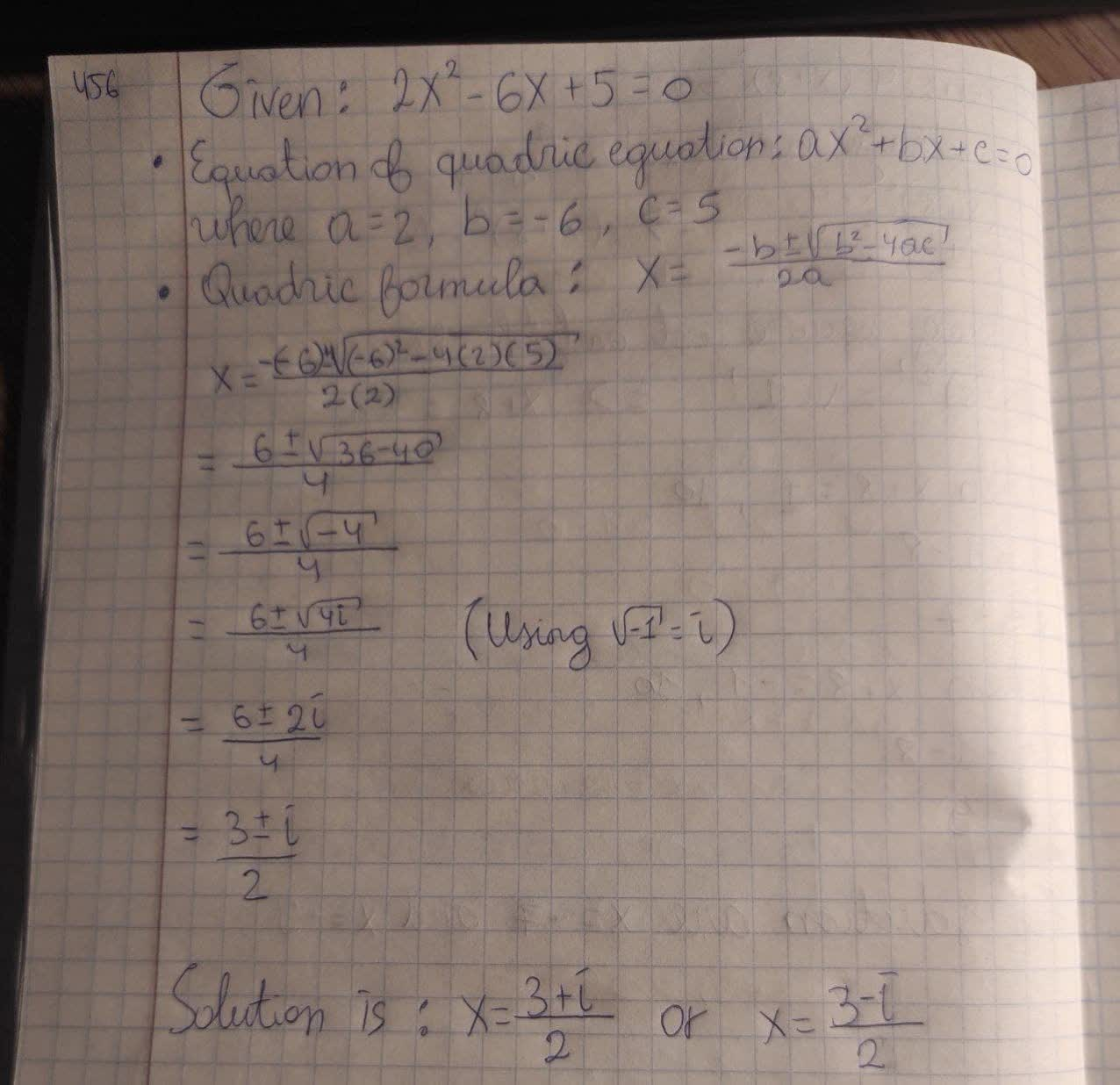Solve the quadratic equation using the quadratic formula. 2x^2 - 6x + 5 = 0Braxton Pugh 2021-02-21 Answered
Solve the quadratic equation using the quadratic formula. $$\displaystyle{2}{x}^{{2}}-{6}{x}+{5}={0}$$

• Questions are typically answered in as fast as 30 minutes

Solve your problem for the price of one coffee

• Math expert for every subject
• Pay only if we can solve itFatema Sutton

Given quadratic equation is $$\displaystyle{2}{x}^{{2}}−{6}{x}+{5}={0}.$$
Standard equation of quadratic equation is $$\displaystyle{a}{x}^{{2}}+{b}{x}+{c}={0}$$.
On comparing the given equation with standard equation of quadratic equation, we get a=2, b=−6 and c=5
$$\displaystyle{x}=\frac{{-{b}\pm\sqrt{{{b}^{{2}}-{4}{a}{c}}}}}{{2}}{a}$$
$$\displaystyle{x}=\frac{{-{\left(-{6}\right)}\pm\sqrt{{{\left(-{6}\right)}^{{2}}-{4}{\left({2}\right)}{\left({5}\right)}}}}}{{2}}{\left({2}\right)}$$
$$\displaystyle=\frac{{{6}\pm\sqrt{{{36}-{40}}}}}{{4}}$$
$$\displaystyle=\frac{{{6}\pm\sqrt{-}{4}}}{{4}}$$
$$\displaystyle={\left({6}\pm\frac{\sqrt{{{4}{i}}}}{{4}}{\left(\sqrt{-}{1}={i}\right)}\right)}$$
$$\displaystyle=\frac{{{6}\pm{2}{i}}}{{4}}$$
$$\displaystyle=\frac{{{3}\pm{i}}}{{2}}$$
Therefore, required solution of given quadratic equation is: x=(3+i)/2 or x=(3-i)/2